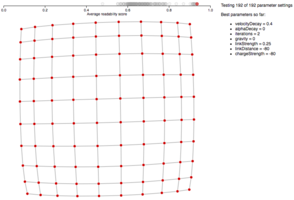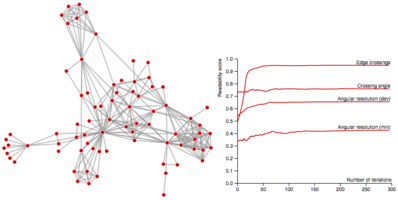I developed an open source library to calculate graph layout quality metrics to help me evaluate graph layout algorithm research (see d3-force-reuse and d3-force-sampled). The metrics are useful for automatically estimating which layout is better, but I also showed a proof of concept using them to automatically find higher quality layouts.

I included four global graph readability metrics, although it could be extended to include additional metrics:

• Edge crossings measures the fraction of edges that cross (intersect) out of an approximate maximum number that can cross.
• Edge crossing angle measures the mean deviation of edge crossing angles from the ideal edge crossing angle (70 degrees).
• Angular resolution (minimum) measures the mean deviation of adjacent incident edge angles from the ideal minimum angles (360 degrees divided by the degree of that node).
• Angular resoluction (deviation) measures the average deviation of angles between incident edges on each vertex.